# Organic Chemistry : Help with Organic Proteins

## Example Questions

### Example Question #275 : Organic Chemistry

Which of the following is an example of a basic amino acid?

Alanine

Leucine

Aspartic acid

Lysine

Tyrosine

Lysine

Explanation:

Lysine's R-group includes an amine (-NH2), which can be ionized by picking up a hydrogen. This ability classifies lysine as basic.

### Example Question #271 : Organic Chemistry

Which of the following amino acids can participate in the formation of a disulfide bridge?

Alanine

Tyrosine

Aspartic acid

Cysteine

Phenylalanine

Cysteine

Explanation:

Cysteine's R-group contains a sulfhydryl group (-SH), which can participate in the formation of a disulfide bridge in a protein's tertiary and/or quaternary structure. Cysteine is the only amino acid to contain a sulfur atom.

### Example Question #276 : Organic Chemistry

Which of the following amino acids is the only one to contain a side chain that results in an achiral amino acid?

Glycene

Alanine

Aspartic acid

Lysine

Glycene

Explanation:

The only achiral amino acid is Glycene. Glycene's side chain is simply a hydrogen. Because a hydrogen already exists on the fundamental structure of an amino acid backbone, a side chain of a single hydrogen atom causes glycene to be achiral.

### Example Question #271 : Organic Chemistry

How many essential amino acids are there?

Twelve

Zero

Ten

Twenty

Nine

Nine

Explanation:

The nine essential amino acids are valine, leucine, isoleucine, histidine, phenylalanine, tryptophan, methionine, threonine, and lysine. These amino acids must be consumed in the diet, since they cannot be synthesized by adult humans.

### Example Question #278 : Organic Chemistry

What is the D/L configuration and the absolute (R/S) configuration of the following amino acid (cysteine)?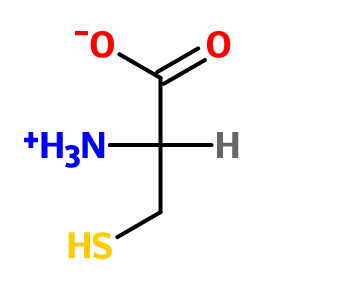Cysteine is achiral

L, R

L, S

D, R

D, S

L, R

Explanation:

Cysteine is an unusual amino acid. Although each of the normal biological amino acids have the L configuration (with the exception of glycine, which is achiral), meaning they can be drawn with the Fischer projection as shown: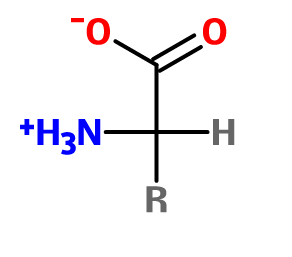the fact that cysteine contains a sulfur group makes the side chain a higher priority than the carboxylic acid. Therefore, the absolute configuration of cysteine will be R, and not S like the other amino acids.

### Example Question #201 : Organic Concepts

Consider L-glutamate, which is shown below.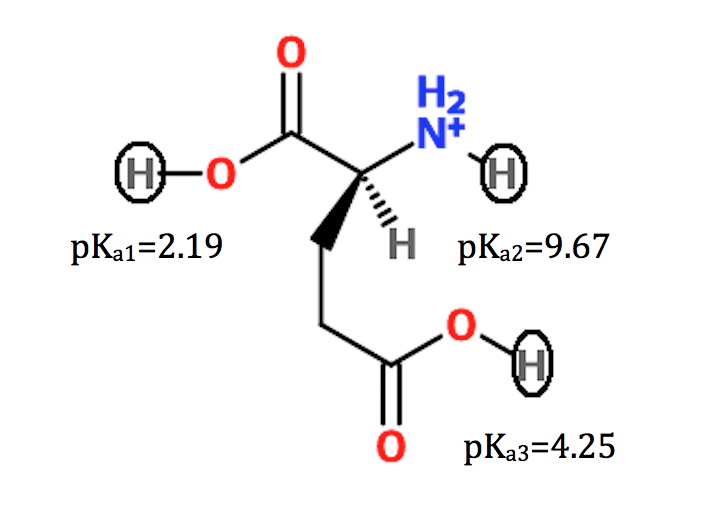Using the pKa information provided, what is the isoelectric point (pI) of the given molecule?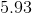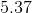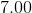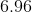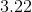Explanation:

The isoelectric point is the average of the two pKa values around which the molecule has an overall neutral charge. In the case of glutamate, the amino acid is in its neutral (zwitterion) form in between the lower two pKa values. Taking the average of the two values gives the pI.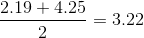### Example Question #1 : Help With Organic Proteins

Consider the amino acid L-lysine, shown below.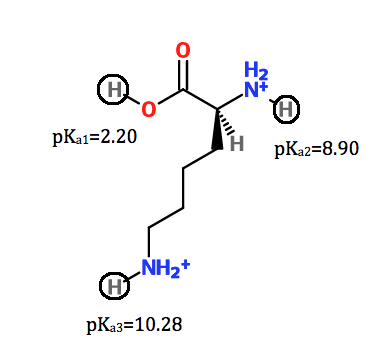Given the pKa values shown, calculate the pI of lysine.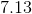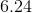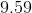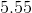Explanation:

The isoelectric point is the average of the two pKa values around which the molecule has an overall neutral charge. In the case of lysine, the amino acid is in its neutral (zwitterion) form in between the higher two pKa values. Taking the average of the two values gives the pI.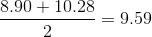### Example Question #31 : Biological Molecules

Treatment of a peptide with Edman's reagent yielded the following phenylthiohydantoin (PTH) derivative: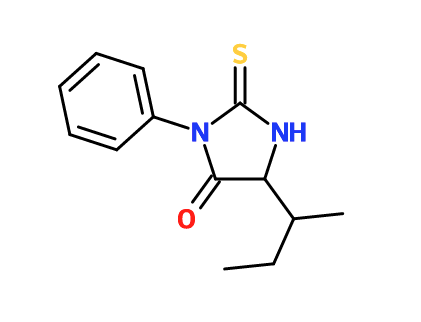What is the side chain of the amino acid at the N-terminus of the peptide?

sec-Butyl

Isobutyl

Amide

Benzyl

Hydrogen

sec-Butyl

Explanation:

Treatment of a peptide with Edman's reagent (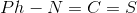) will remove the N-terminal amino acid. The PTH derivative will look as follows: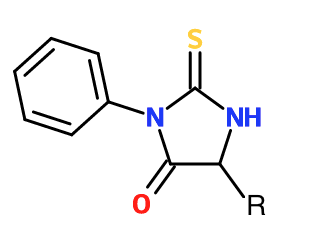In the molecule in the question, a sec-butyl replaces the R group. This is the amino acid isoleucine.

### Example Question #34 : Biological Molecules

What is the amino acid that is synthesized from the malonic ester synthesis shown?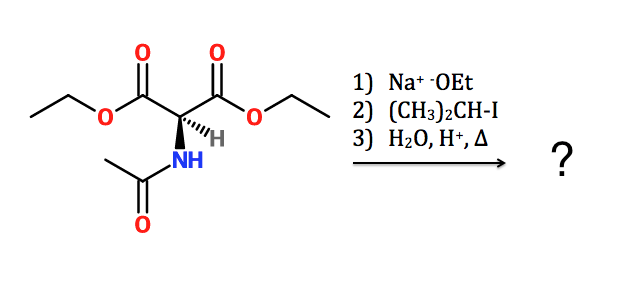Alanine

Lysine

Leucine

Isoleucine

Valine

Valine

Explanation:

First step: Deprotonation of alpha hydrogen and formation of the malonic ester enolate.

Second step: bimolecular nucleophilic substitution, resulting in addition of the isopropyl group to the alpha carbon.

Third step: hydrolysis of amide and ester bonds, followed by decarboxylation

The synthesis forms valine, because the alkyl halide that is attacked is an isopropyl group (Valine's side chain is an isopropyl group). A more detailed mechanism is shown below.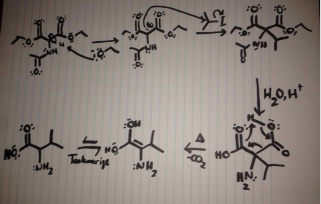### All Organic Chemistry Resources